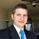203 views
Based on science formula , momentum = mass* velocity
here mass = volume , and velocity here is average velocity .
For VOLUME , MOMENTUM , VOLATILITY = it gives accurate value ( not slow like bb and ma )
Release Notes: we all know , momentum scientific formula = mass * speed ,
come to the point ,
volume = mass , speed = average speed direction of traders .

WHY THIS INDICATOR IS SO POWERFUL AND USEFUL ?

volume indicator only tells the number of traders entering in market ,right ?
but this indicator tell the number of trader trading in which direction .